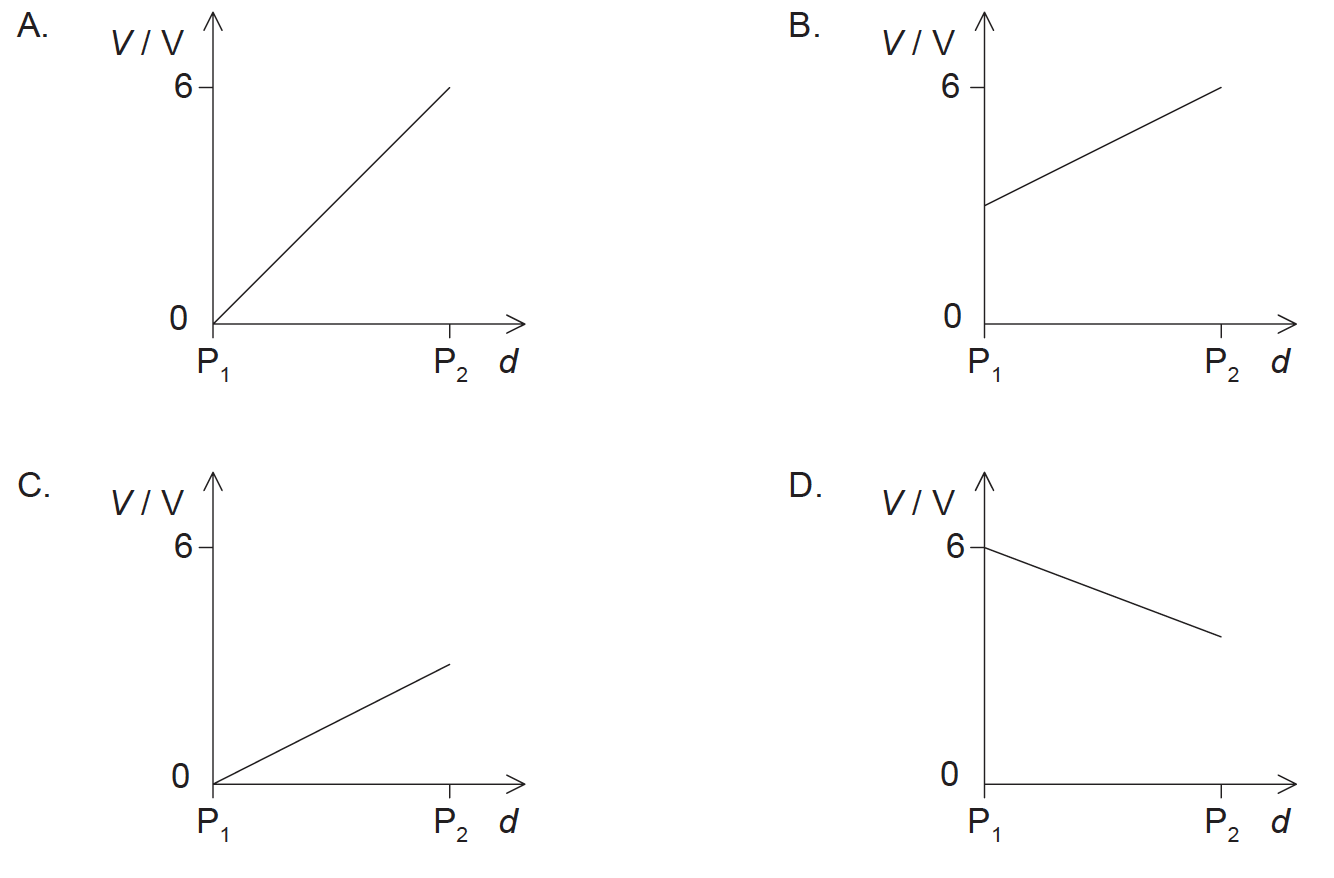1. A cell of emf 6.0 V and negligible internal resistance is connected to three resistors as shown.

The resistors have resistance of 3.0 Ω and 6.0 Ω as shown.What is the current in resistor X?

2. A filament lamp and a semiconducting diode have the voltage–current

(V–I) characteristics shown and are connected in parallel.What is the resistance of the lamp and the resistance of the diode when the current in each device is 2.0 A?3. Four point charges of magnitudes +q, +q, −q, and −q are held in place at the corners of a square of side r.The Coulomb constant is k. Which of the following is the electrical potential at the centre of the square O?

4. When an electric cell of negligible internal resistance is connected to a resistor of resistance 4R, the power dissipated in the resistor is P. What is the power dissipated in a resistor of resistance value R when it is connected to the same cell?
5. A circuit is formed by connecting a resistor between the terminals of a battery of electromotive force (emf) 6 V. The battery has internal resistance. Which statement is correct when 1 C of charge flows around the complete circuit?
6. An ion of charge +Q moves vertically upwards through a small distance s in a uniform vertical electric field. The electric field has a strength E and its direction is shown in the diagram.What is the electric potential difference between the initial and final position of the ion?

7. A 12V battery has an internal resistance of 2.0Ω. A load of variable resistance is connected across the battery and adjusted to have resistance equal to that of the internal resistance of the battery. Which statement is correct for this circuit?
8. A proton p is at rest between the poles of two horizontal magnets as shown below.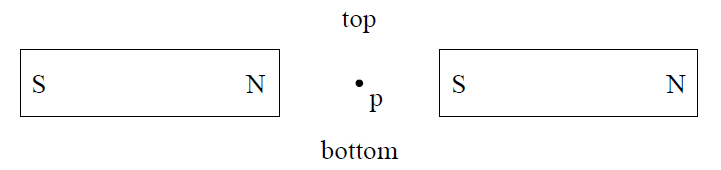The magnetic force on the proton is

9. The diagram shows the path of a particle in a region of uniform magnetic field. The field is directed into the plane of the page.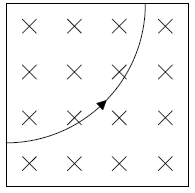This particle could be

10. A current carrying wire is in the same plane as a uniform magnetic field. The angle between the wire and the magnetic field is θ.The magnetic force on the current carrying wire is

11. Electrons, each with a charge e, move with speed v along a metal wire. The electric current in the wire is I.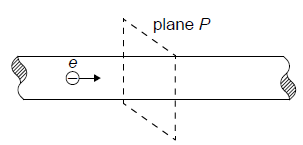Plane P is perpendicular to the wire. How many electrons pass through plane P in each second?

12. An ion follows a circular path in a uniform magnetic field. Which single change decreases the radius of the path?
13. A resistor has a resistance R. The potential difference across the resistor is V. Which of the following gives the energy dissipated in the resistor in time t?
14. The electric potential is

VR at a point R in an electric field and at another point S the electric potential is VS. Which of the following is the work done by the electric field on a point charge +q as it moves from R to S?

15. Two wires, X and Y, are made from the same metal. The wires are connected in series. The radius of X is twice that of Y. The carrier drift speed in X is

vX and in Y it is vY.

What is the value of the ratio (vX)/(vY)?

16. A copper wire with length L and radius r has a resistance R.

What is the radius of a copper wire with length L/2 and resistance R?

17. Positive charge is uniformly distributed on a semi-circular plastic rod. What is the direction of the electric field strength at point S?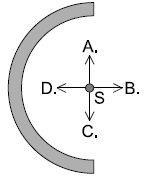18. Two resistors, of resistance

R1 and R2, are connected in series with a cell of emf ε and negligible internal resistance.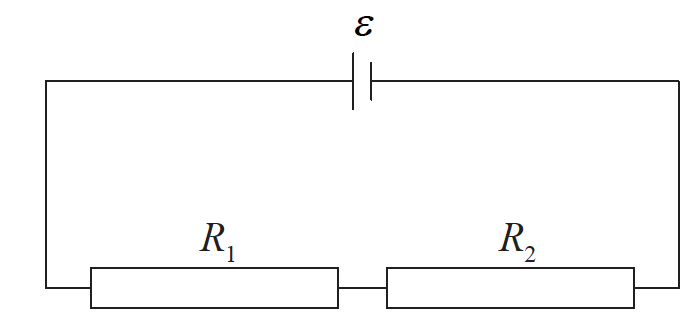Which expression gives the potential difference across the resistor of resistance R1?

19. A voltmeter of resistance 50kΩ is used to measure the electric potential difference in a circuit, as shown. The cell has an electromotive force (emf) of 5.0V and negligible internal resistance.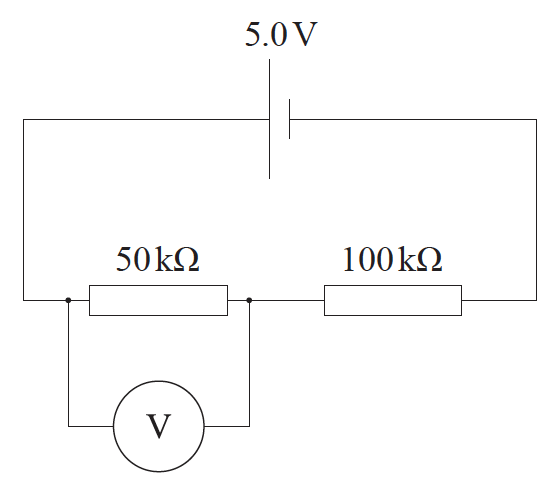What is the reading on the voltmeter?

20. A positively charged particle follows a circular path as shown below.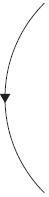Which of the following electric fields could have caused the charged particle to follow the above path?

21. A metal rod M is falling vertically within a horizontal magnetic field. The metal rod and magnetic field are directed into the paper. What is the direction of the initial force acting on the metal rod that is predicted by Lenz’s law?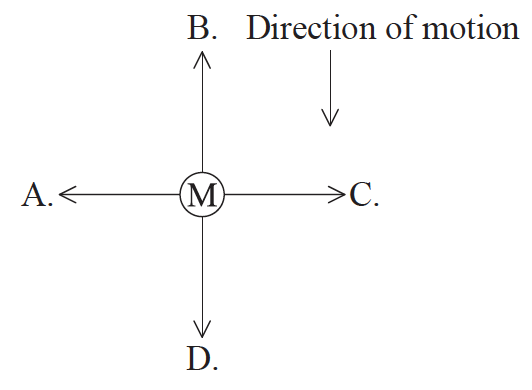22. A circuit consists of a cell of electromotive force (emf) 6.0V and negligible internal resistance connected to two resistors of 4.0Ω.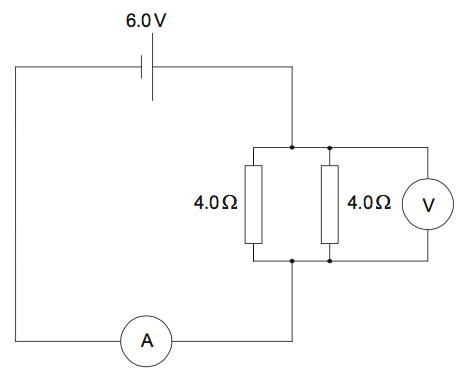The ammeter has resistance equal to 1.0Ω and the voltmeter is ideal. What are the readings of the ammeter and the voltmeter?23. Two isolated point charges,

-7μC and +2μC, are at a fixed distance apart. At which point is it possible for the electric field strength to be zero?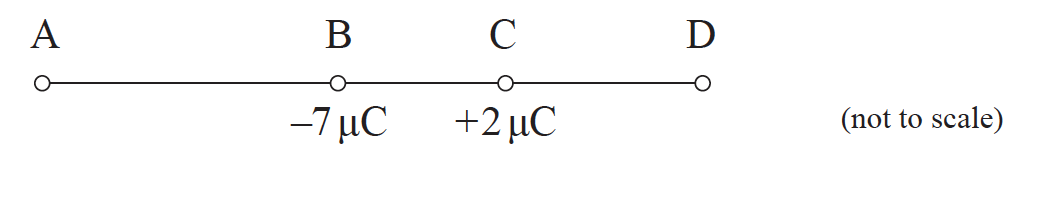24. An ideal ammeter is used to measure the current in a resistor. Which of the following gives the resistance of an ideal ammeter and the way it is connected to the resistor?25. The diagram below shows a uniform electric field of strength E. The field is in a vacuum.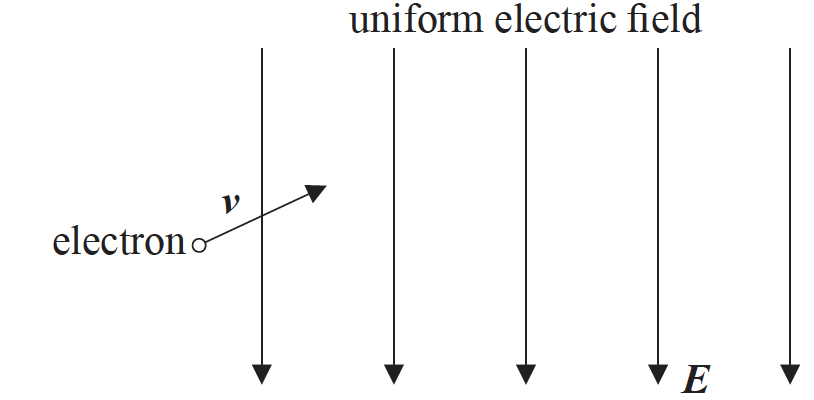An electron enters the field with a velocity v in the direction shown. The electron is moving in the plane of the paper. The path followed by the electron will be

26. A long straight wire carries an electric current perpendicularly out of the paper. Which of the following represents the magnetic field pattern due to the current?
27. The diagram shows the magnetic field surrounding two current-carrying metal wires P and Q. The wires are parallel to each other and at right angles to the plane of the page.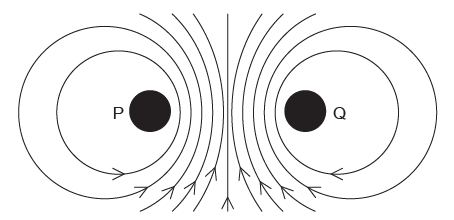What is the direction of the electron flow in P and the direction of the electron flow in Q?28. A lamp is connected to an electric cell and it lights at its working voltage. The lamp is then connected to the same cell in a circuit with an ideal ammeter and an ideal voltmeter. Which circuit allows the lamp to light at the original brightness?
29. The diagram shows an electric circuit containing a potentiometer of maximum resistance R. The potentiometer is connected in series with a resistor also of resistance R. The electromotive force (emf) of the battery is 6 V and its internal resistance is negligible.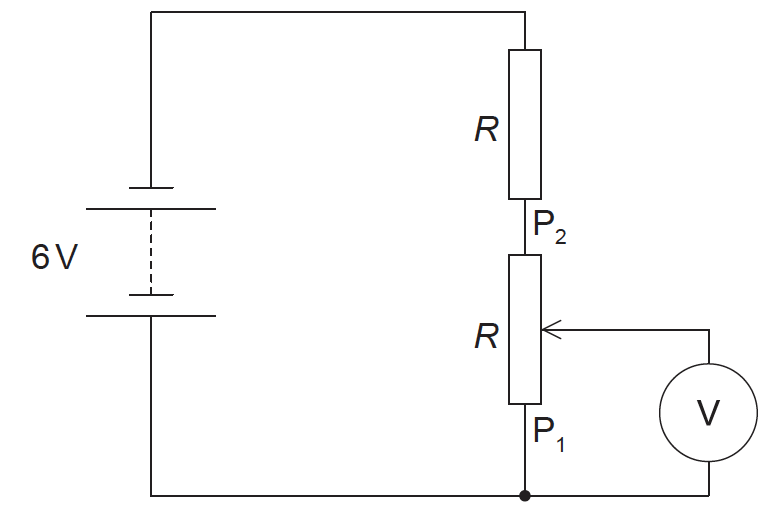The slider on the potentiometer is moved from P1 to P2. Which graph shows the variation of the voltmeter V reading with slider distance d?